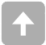## 一、需求确定

### 1.1、总体需求

超级电容模块作为电源到底盘电机中间的功率控制模块，要求完成以下任务：
1、限制电源的输出功率，做到功率实时监控和动态调整。
2、利用电机组功率消耗的谷值时间给超级电容组充电，以达到能量合理分配和利用的目的。
3、实时汇报给操作手超级电容当前电量，方便操作手进行调整。

### 1.2、具体需求

电路主要分为三个大类：1、电源线路 2、检测线路 3、控制电路。
1、电源线路
Ⅰ.BUCK降压转换器：通过控制转换器输出电压来限制输出功率，达到控制输入功率目的（必需）
Ⅱ.BOOST升压转换器：将电源升压给超级电容充电（必需）
Ⅲ.各个芯片的供电电源模块（必需）
2、检测线路
Ⅰ.电流检测模块：总的输入和输出电流（必需）
Ⅱ.电压检测模块：输入电压，输出电压和超级电容电压（必需）
3、控制电路

Ⅰ.计算输入和输出功率（必需）
Ⅱ.使用输入功率与当前等级的限制功率比较，计算BUCK转换器的PWM的占空比（必需）
Ⅲ.使用电容电压计算其剩余电量，发送给操作手，由他控制超级电容放电（必需）
Ⅳ.使用输出功率与一定值作比较，和电容电压共同控制超级电容的充电（必需）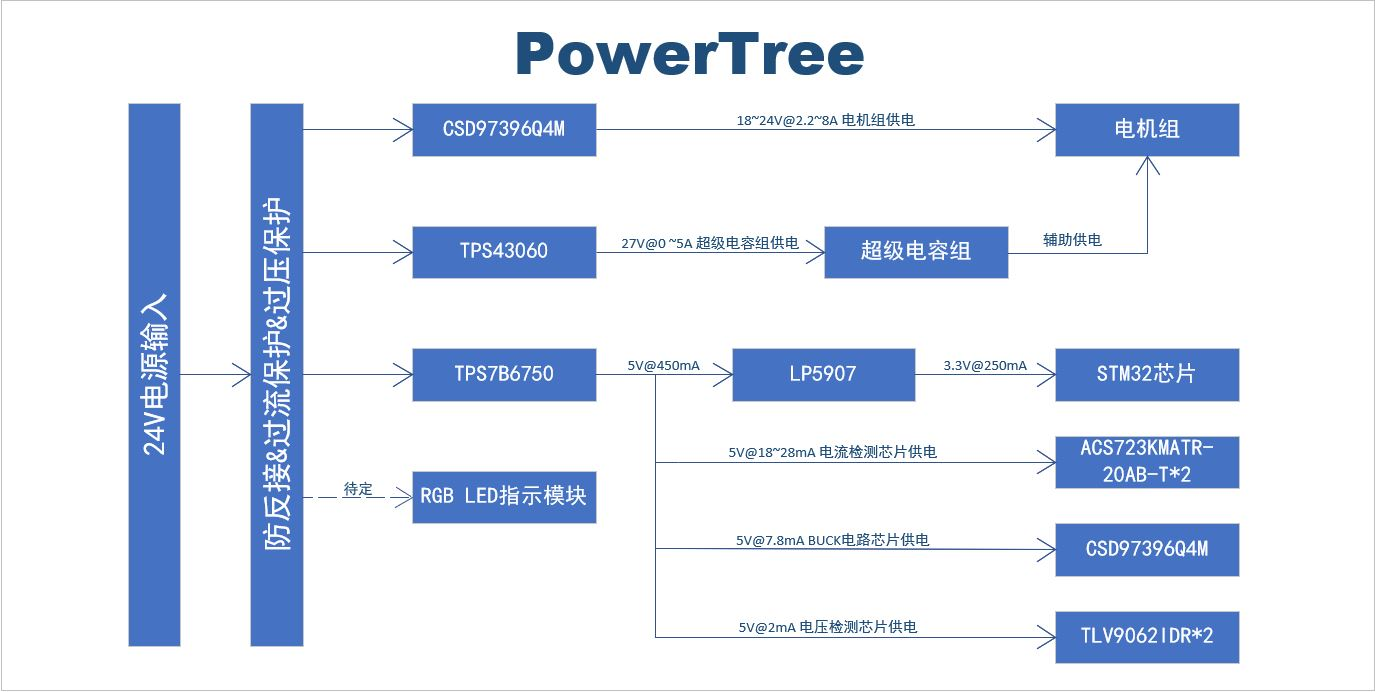## 二、电路参数实际计算

### 2.1、效率分析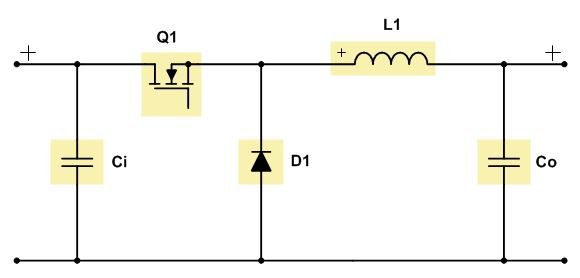测试电路采用非同步BUCK电路，输出电压值18~24V，测试频率1kHz，负载连接一个无刷直流电机，预计转换效率在95%左右(仿真结果)。实际转换效率75%左右，同时电压有明显波动，但对电机的运动未造成巨大影响（也可能受实验参数限制，未观察出影响）。
分析：由于测试所使用电路相当简单，不存在电路本身结构造成功率损失的问题。于是将问题矛头指向MOS管，二极管和电感这三个元件造成的损耗。测得MOS管IRFP250N的 $R_{DS(ON)}$ 在75mΩ左右，二极管10A10的 $V_F$ 最大为1V，铁氧体芯电感(双1.2线，10mH)的DCR在110mΩ左右。

MOS管在1KHz频率下的主要损耗为：

$P_D = P_{CON} + P_{SW\_OPEN} + P_{SW\_CLOSE} (2-1-1)$

$P_{CON}$ 的计算通式为（取占空比的中间值的导通损耗为准）：

$P_{CON} = I_{DS}^2 \ast R_{DS(ON)} \ast D = 1.75^2 \times 0.075 \times 0.875 \approx 0.1148W (2-1-2)$

$P_{SW\_OPEN}$

$P_{SW\_CLOSE}$的计算过程若是较真起来会相当复杂，这里先采用近似最坏假设计算：

$\begin{equation}\begin{split} P_{SW\_OPEN}&= \frac 12 \ast V_{DS} \ast I_{DS} \ast (T_r + T_{d(ON)})\\ &\frac 12 \times 0.075 \times 1000 \times (14 + 43) \times 10^{-9} = 0.0065mW\\ \end{split}\end{equation} (2-1-3)$

$\begin{equation}\begin{split} P_{SW\_CLOSE}&= \frac 12 \ast V_{DS} \ast I_{DS} \ast (T_f + T_{d(OFF)})\\ &\frac 12 \times 0.075 \times 1000 \times (14 + 33) \times 10^{-9} = 0.0085mW\\ \end{split}\end{equation} (2-1-4)$

$P_D = P_{CON} + P_{SW\_OPEN} + P_{SW\_CLOSE} = 0.1148 + (0.0065 + 0.0085) \times 10^(-3) \approx 0.1148W (2-1-5)$

$P_D = V_F \ast I_F \ast (1 - D) = 1 \times 1.75 \times (1 - 0.75) = 0.4375W (2-1-6)$

$P_{Cu} = I_F^2 \ast DCR = 1.752 \times 0.11 \approx 0.3369W (2-1-7)$

$励磁B_{PP}^{+} = \frac {(U_i - U_o) \ast D \ast T}{N \ast A_e} = \frac {(24 - 21) \times 0.875 X 1 \times 10^{-3}}{28 \times (31 - 19) \times 13 \times 10^{-4}} \approx 6.0096 \times 10^{-3}Wb (2-1-8)$

$退磁B_{PP}^{-} = \frac {U_o \ast (1 - D) \ast T}{N \ast A_e} = \frac {21 X 0.125 \times 1 \times 10^{-3}}{28 \times (31 - 19) \times 13 \times 10^{-4}} \approx 6.0096 \times 10^{-3}Wb (2-1-9)$

$P_{Fe} = B_{PP}^{2} \ast f = (6.0096 \times 10^{-3})^2 \times 1000 \approx 0.0361W (2-1-10)$

$B_{PP}$ 为磁通变化量，D为占空比，T为周期，N为匝数， $A_e$ 为磁芯横截面积， $U_i$ ， $U_o$ 分别为输入电压和输出电压，f为频率

$P_D = P_{Cu} + P_{Fe} = 0.3369 + 0.0361 = 0.3730W (2-1-11)$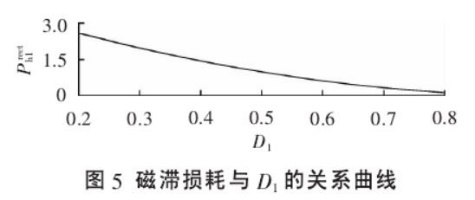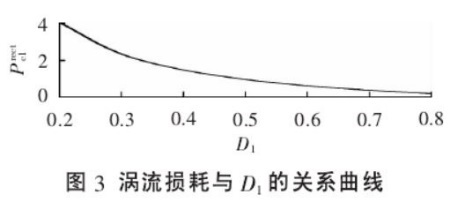### 2.2、主要参数计算

1、将电路替换为同步BUCK电路。控制难度会有所提升，但采用TI公司的一些器件可以达到稳定控制。（值得注意的是，同步电路并非完美，它在轻负载的情况下造成了不良的电路反应，即在高侧MOS管关断时，电感电流可能会倒流，但使用二极管不存在该问题，因此我选用的芯片有二极管仿真模式，价格也相对合理。）
2、提高开关频率，可以减小电感体积，提高能量密度，改善动态响应。（换用外径20mm的电感，原来的为31mm。）
3、采用软开关技术，减小由提高频率带来的开关损耗增加的问题。（尚未考虑周全，且计划先提升至10kHz，不算高频。）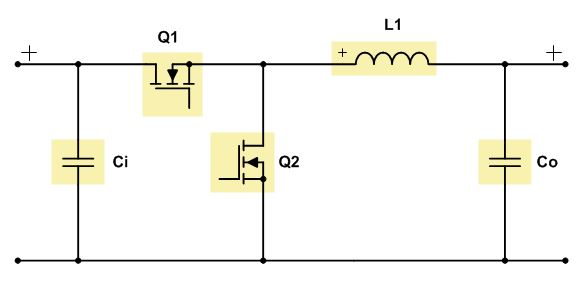DC-DC转换电路外围电感选型需要考虑以下几个参数：

#### 电感

$∆I_L = \frac {(U_i - U_o) \ast D}{L_{临界} \ast f} = I_{out} \ast r (2-2-1)$

r一般在 0.3~0.5 之间。这里，我引入了一个重要的参数电流纹波系数r。为什么在算电感量之前要引入该参数？因为我要明确告诉后来人，这个参数远比电感值本身重要。

$r = \frac {∆I}{I_L} \equiv 2 \times \frac {I_{AC}}{I_{DC}} (2-2-2)$

$u = L \ast \frac {d_i}{d_t} (2-2-3)$

$r = \frac {∆I}{I_L} = \frac {E \ast t}{L \ast I_L } \equiv \frac {V_{ON} \ast D}{L \ast I_L \ast f} \equiv \frac {V_{OFF} \ast (1-D)}{L \ast I_L \ast f} (2-2-4)$

$L = \frac {V_{ON} \ast D}{r \ast I_L \ast f} 或 L \ast I_L = \frac {E \ast t}{r} (2-2-5)$

$L_{min} = \frac {U_{i\_{MAX}} - U_o \ast D_{max}}{I_L \ast r \ast f} = \frac {(25-18) \times 18}{2.2 \times 0.3 X 23.5 \times 10 \times 10^3} \approx 1.219mH (2-2-6)$

$I_{Lpeak} = I_L \ast (1 + \frac r2) = 7 \times (1 + \frac {0.2}{2}) = 7.7A (2-2-7)$

$L_{chosen} = \frac {L_{min}}{0.8 \times 0.75} = \frac {1.219}{0.8 X 0.75} \approx 2.032mH (2-2-8)$

$P_{Cu} = I_Z^2 \ast DCR = 5^2 \times 11.55 = 288.75mW (2-2-9)$

$P_{Fe} = B_{PP}^2 \ast f = (14.9148 \times 10^{-3})^2 \times 10 ^4 \approx 2.2245W (2-2-10)$

$P_D = P_{Cu} + P_{Fe} = 0.28875 + 2.2245 =2.5133W (2-2-11)$

#### 电容

$C_o$ 很大的话，可以保证输出电压近似恒定，但是$C_o$很大会导致体积和成本更大。因此实际中根据容许的输出电压纹波来选择 $C_o$ 的值。设计时总是按照电感电流谐波全部进入 $C_o$ ，恒定分量进入负载（如果带阻性负载，在闭环电路的控制下，输出电压恒定，确实是这样的）。电感电流i的谐波进入电容，由电容的寄生电阻ESR、寄生电感ESL，和$C_o$值决定电压纹波的。 对于低于500kHz的谐波，ESL产生的电压纹波可以忽略。因此，输出电容中由ESR和 $C_o$ 决定纹波电压分量。由ESR产生的纹波分量正比于电感电流纹波分量，由 $C_o$ 决定的纹波分量与流过电容的电流积分成正比。这两个分量相位是不同的。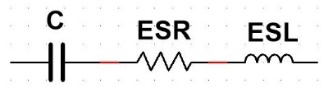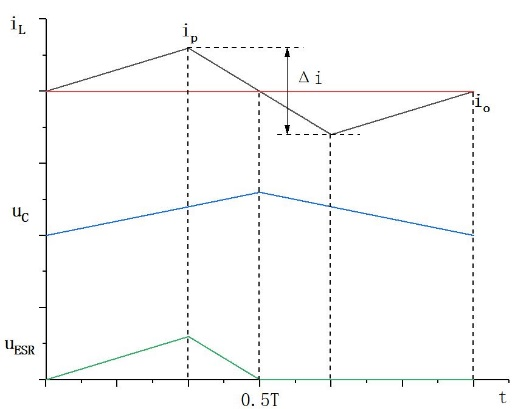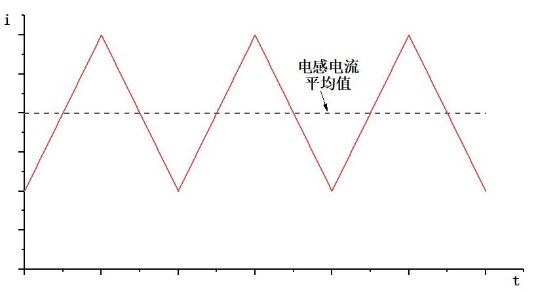（1）最大输出纹波峰峰值小于输出电压的1%，即

$V_{o\_RIPPLE\_MAX} = 1% \ast U_{o\_{MAX}} = 1% \times 23.5 = 0.235V$

（2）负载突增时，可接受的最大电压下垂量为： $∆V_{DROP} = 1.175V$ ;
（3）负载突增时，可接受的最大超调量为： $V_{OVERSHOOT} = 1.175V$ ;

$r_{Ui\_{MAX}} = \frac {U_{i\_{MAX}} - U_o \ast D_{max}} {I_o \ast L_{chosen} \ast f} = \frac {(25 - 18) \times 18}{2.2 \times 2 \times 10^{-3} \times 23.5 \times 10 X 10^3} \approx 0.12 （2-2-12）$

$I_{o\_RIPPLE\_MAX} = I_o \ast r_{Ui\_MAX} = 2.2 \times 0.12 = 0.2640A （2-2-13）$

$ESR \le \frac {V_{o\_RIPPLE\_MAX}}{I_o \ast r_{Ui \_ MAX}} = \frac {0.235}{2.2 \times 0.12} = 0.8902Ω （2-2-14）$

$∆S = \frac {1}{8f} \ast I_{o \_ RIPPLE \_ MAX} （2-2-15）$

$∆Q = C_o \ast V_{o \_ RIPPLE \_ MAX} （2-2-16）$

$C_{o\_1} \ge \frac {1}{8f} \ast \frac {I_{o\_RIPPLE\_MAX}}{V_{o\_RIPPLE\_MAX}} = \frac {1}{8 \times 10^4} \times \frac {2.2×0.12}{0.235} = 14.0425μF （2-2-17）$

$C \ge \frac {I_o \ast ∆t}{ V_{o\_RIPPLE\_MAX}} = \frac {2 \ast T \ast I_o}{∆V_{DROP}} = \frac {2 I_o}{∆V_{DROP} \ast f} （2-2-18）$

$C_{o\_2} \ge \frac {2 \ast ∆I_o}{∆V_{DROP} \ast f} = \frac {2 \times \frac 52}{1.175 \times 10^4} = 425.5319uF （2-2-19）$

$\frac 12 \ast C \ast (V_x^2 - V_o^2) = \frac 12 \ast L \ast I_o^2 （2-2-20）$

$C\ge \frac {L \ast I_o^2}{(V_x + V_o ) \ast (V_x - V_o )} \approx \frac {L \ast I_o^2}{2 \ast V_o \ast ∆V_{OVERSHOOT}} （2-2-21）$

$C_{o\_3} \ge \frac {2 \times 10^(-3) \times 5^2}{2 \times 24 \times 1.175} \approx 425.5319uF （2-2-22）$

$I_{Co\_RMS\_MAX} = I_o \ast \frac {r_{Ui\_MAX}}{\sqrt {12}} = 2.2 \times \frac {0.12}{\sqrt {12}} = 76.2102mA （2-2-23）$

$I_{Co\_RMS\_MIN} = I_o \ast \frac {r_{Ui\_MIN}}{\sqrt {12}} = 2.2 \times \frac {0.096}{\sqrt {12}} = 60.9682mA （2-2-24）$

$P_{Co\_MAX} = I_{Co\_RMS\_MAX}^2 \ast ESR = 76.2102^2 \times 0.05 = 0.2904mW （2-2-25）$

$P_{Co\_MIN} = I_{Co\_RMS\_MIN}^2 \ast ESR = 60.9682^2 \times 0.05 = 0.1859mW （2-2-26）$

$V_{RIPP\_PP\_MAX} = 2% \ast U_{i\_MAX} = 2% \times 25 = 0.5V （2-2-27）$

$D_{IDEAL\_Ui\_MAX} = \frac {U_O}{U_{i\_MAX}} = \frac {18}{25} = 0.72 （2-2-28）$

$\begin{equation}\begin{split} C_i&= \frac {I_o \ast D_{IDEAL\_Ui\_MAX} \ast (1-D_{IDEAL\_Ui\_MAX})}{f \ast (V_{RIPP\_PP\_MAX} - ESR \ast I_o \ast (1 + (0.5 \ast r_{Ui\_MAX})))}\\ &= \frac {2.2 \times 0.72 \times (1-0.72)}{10^4 \times (0.5 - 0.05 \times 2.2 \times (1 + (0.5 \times 0.12)))} \approx 0.0065mW\\ \end{split}\end{equation} (2-2-29)$

$\begin{equation}\begin{split} I_{Ci\_RMS\_MAX}&= I_o \ast \sqrt {D_{IDEAL\_Ui\_MAX} \ast (1-D_{IDEAL\_Ui\_MAX} + \frac {r_{Ui\_MIN}^2}{12})}\\ &= 5 \times \sqrt {0.96 \times (1-0.96 + \frac {0.015^2}{12})} = 0.9800A\\ \end{split}\end{equation} (2-2-30)$

$\begin{equation}\begin{split} I_{Ci\_RMS\_MIN}&= I_o \ast \sqrt {D_{IDEAL\_Ui\_MIN} \ast (1-D_{IDEAL\_Ui\_MIN} + \frac {r_{Ui\_MAX}^2}{12})}\\ &= 2.2 \times \sqrt {0.7660 \times (1-0.7660 + \frac {0.12^2}{12})} = 0.9338A\\ \end{split}\end{equation} (2-2-31)$

$P_{Ci\_MAX} = I_{Ci\_RMS\_MAX}^2 \ast ESR = 0.9800 ^2 \times 0.05 = 48.0200mW （2-2-32）$

$P_{Ci\_MIN} = I_{Ci\_RMS\_MIN}^2 \ast ESR = 0.9338^2 \times 0.05 = 43.5991mW （2-2-33）$

 周岩,张俊波,陈麒米.开关变换器功率电感磁损建模及应用[J]. 电力自动化设备,2017,37(11):132-135.
 马文超. BUCK电路外围器件参数选型分析[J]. 电子与封装,2019,19(12):30-31.
 Sanjaya Maniktala.Switching Power Supplies A-Z,2E[M]. Beijing: The Posts and Telecommunications Press,2015:47-80.